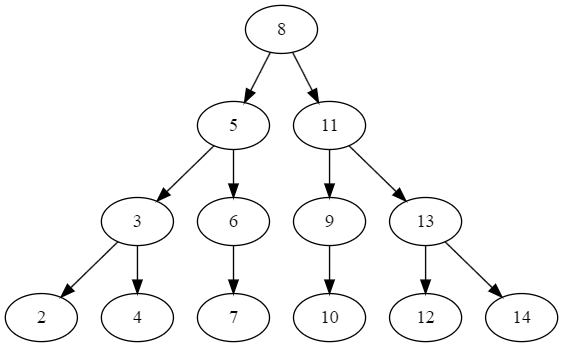有序数组连续计算中位数

2016/04/22

1. 获取总长度, 并根据首尾的刻度值, 计算中间可取整的刻度值, 如 1 - 78 中间, 可按 10 取整, 即 10, 20, 30 … 70 这样.
2. 设一个刻度总和的变量 `sum`, 试探性的绘制位于中间的刻度, 并计算该刻度(字符串)在画布上的长度, 将该长度累加至 `sum`, 并与总长度比较.
1. 如果小于总长度, 绘制该刻度, 并借助首尾刻度, 以及该刻度, 继续求出两个刻度值. (位于首刻度与该刻度中间, 以及该刻度与尾刻度中间). 递归进入上一步骤.
2. 如果大于总长度, 则退出.``````#include <vector>
#include <queue>
#include <iostream>

std::vector<int> GetMedianList(int min, int max)
{
if (min > max)
return {};

std::vector<int> vecRst;
std::queue<std::pair<int, int>> q;
q.push(std::make_pair(min, max));
do
{
auto range = q.front(); q.pop();
int mid = (range.first + range.second) / 2;
if (mid != range.first)
vecRst.push_back(mid);
else
continue;
q.push(std::make_pair(range.first, mid));
q.push(std::make_pair(mid, range.second));
} while (!q.empty());

return vecRst;
}

int main()
{
for (int i : GetMedianList(2, 15))
{
std::cout << i << " ";
}
std::cout << std::endl;
}
``````